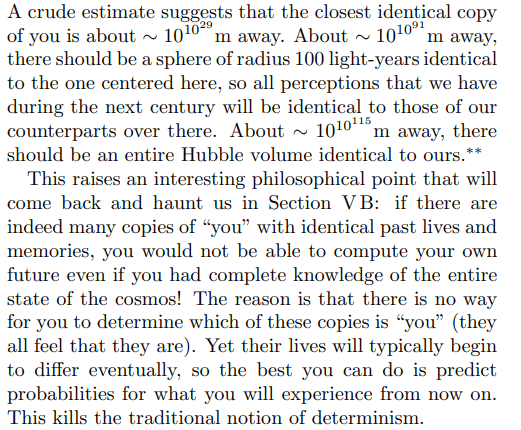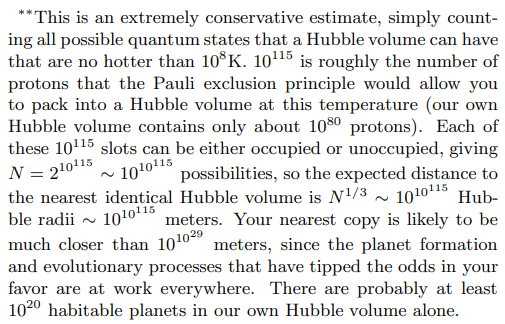# The distance to the nearest identical Hubble volume in light years?

• B
• Cerenkov
Is there any science to this? Or are we arguing philosophy? Is a world identical to ours except that you asked for a cheesebrurger last week "close enough"? This seems more like a topic for late-night undergraduate musings than something subject to scientific inquiry.

•PeroK
Can you address the part about quantum repeats? Is the wavefunction of particle positions supposed to be infinite, and, if so, even in an infinite universe, can every possibility truly repeat in that case?
Remember we are talking about repeats of our observable universe, which is finite in size. Any "wave functions" we use in a quantum model would be restricted to that finite volume. And in that finite volume there are only a finite number of possible quantum states. That is the basis for the "repeat" claim.

it is true that odds are you'd probably encounter countless numbers of different Earths with alternate histories on the way to finding the identical Earth.
I don't know that anyone has actually calculated this, but it seems plausible.

Well, to be an identical Earth, it would have to send an explorer out searching for an identical Earth in the same way you went out searching. Does that mean you'd cross paths?
No, because the identical explorer to you from "repeat Earth" would be going away from you. You are setting off from Earth towards repeat Earth. The repeat Earth explorer would be setting off from repeat Earth towards repeat repeat Earth, in the opposite direction from Earth.

And does that mean the path to and from each Earth would be mirror images?
No, because that would mean the explorer was not from repeat Earth, but from a different almost-copy of Earth where he set out in the opposite direction, towards Earth instead of towards repeat repeat Earth.

This seems more like a topic for late-night undergraduate musings than something subject to scientific inquiry.
Was thinking how this sounds like a vsauce episodeDoesn't a particle have a chance to be found at any distance toward infinity? So if the universe is infinite, and no matter how infinitely large, any particle on a repeat Earth can instead be found at the boundaries of infinity, so that a certain exact match could be mathematically impossible. Perhaps.

any particle on a repeat Earth can instead be found at the boundaries of infinity
The boundaries of infinity is an oxymoron.

•syfry
The boundaries of infinity is an oxymoron.
Of course, but all I'm saying is that whatever size area we choose as possible for Earth to repeat, any of its particles can appear beyond that area.

So the problem with any absolute replication down to the particle layer is that any of the components can be found outside of the replication, to the farthest reaches of infinity.

If I understand correctly the probability of where to find any particle.

•Jaime Rudas
No, because that would mean the explorer was not from repeat Earth, but from a different almost-copy of Earth where he set out in the opposite direction, towards Earth instead of towards repeat repeat Earth.
This is where, IMO, the repeat Earth theory loses touch with the pure mathematics on which it relies. All you can do is find a planet that is identical to Earth in every respect at a given point in time. But, such a planet will immediately (almost certainly) diverge from Earth as microscopic interactions produce different outcomes from the same initial conditions. And, you don't know which repeat Earth will still be a repeat Earth one second later.

To put in mathematical terms. The following statement is false:$$\exists \ P: \forall t: \Psi_P(t) = \Psi_E(t)$$Where ##E## is planet Earth and ##P## is some planet. But, the following statement could be true:$$\forall t, \exists \ P: \Psi_P(t) = \Psi_E(t)$$Or, to put it another way, there is no time-independent identical Earth:$$\forall P, \exists t: \Psi_P(t) \ne \Psi_E(t)$$In general, if we want to invoke the paradoxes of infinite sequence, we cannot pick and choose the subtlties of infinite sequences that suit some narrative.

To go back to the original calculation. What that says is that for a given ##t## we would expect to find an identical Earth (let's stick to Earth rather than an entire Hubble volume!) within a distance ##d##. But, the probability that the "identical" Earth is still identical one second later is practically zero. Instead, if you believe the theory, another planet within that distance that was previously nearly identical to Earth is now identical to Earth. The key point is that the planets identified as identical Earths are changing all the time.

That said, the whole thing is fantasy rather than real physics. All of this is completely untestable.

•syfry and Jaime Rudas
Maybe it's easier to understand if one stated there are an infinite amount of Earths exactly like our own at all times of history and evolution. (In which case it is conjecture and risks the thread being closed.)

All you can do is find a planet that is identical to Earth in every respect at a given point in time.
You will find that, yes, but in a spatially infinite universe, if you look further, you will find, not just an Earth, but an entire observable universe, that matches ours through its entire history. (I'm not clear on whether Tegmark also claims that the entire future will be the same.)

All of this is completely untestable.
Agreed.

Doesn't a particle have a chance to be found at any distance toward infinity?
No. Here you are using a very simple concept of a wave function in non-relativistic QM. But the cosmological models being used in the argument discussed in this thread are based on relativistic quantum field theory, where the statement you are making can't even be formulated as you state it. Non-relativistic QM doesn't even cover this domain. The key statement in QFT for the argument is that, within a finite volume, there are only a finite number of possible states. AFAIK that statement is valid in QFT.

You will find that, yes, but in a spatially infinite universe, if you look further, you will find, not just an Earth, but an entire observable universe, that matches ours through its entire history.
And this would be valid for any given moment in the future.

And this would be valid for any given moment in the future.
Everything could be exactly the same except a blade of grass is missing from the Browns/Steelers game (non-scientific, I know, sorry)

The Wiki gives a reference to the Tegmark estimate paper,
1. Tegmark, Max (2003). "Parallel Universes". Scientific American. 288 (5): 40–51. arXiv:astro-ph/0302131. Bibcode:2003SciAm.288e..40T. doi:10.1038/scientificamerican0503-40. PMID 12701329.
from the PDF, Tegmark's words are,Just an estimate using the Pauli exclusion principle.
Low balled, and not repeatable, ie one doesn't find another 'earth ' every set of this distance - could be less, could be more depending again upon chance. [ ie there could be an exact earth in the sphere just outside our own - whose to know ]

••syfry, PeroK and Ibix
The Wiki gives a reference to the Tegmark estimate paper,
1. Tegmark, Max (2003). "Parallel Universes". Scientific American. 288 (5): 40–51. arXiv:astro-ph/0302131. Bibcode:2003SciAm.288e..40T. doi:10.1038/scientificamerican0503-40. PMID 12701329.
from the PDF, Tegmark's words are,
View attachment 332312
View attachment 332313

Just an estimate using the Pauli exclusion principle.
Low balled, and not repeatable, ie one doesn't find another 'earth ' every set of this distance - could be less, could be more depending again upon chance. [ ie there could be an exact earth in the sphere just outside our own - whose to know ]
Here's a key question. We have a statement:

In a region of the universe of radius ##10^{10^{115}} \ m## there is another Hubble volume identical to the Hubble volume centred on the Earth.

Is Tegmark saying:

a) That statement is definitely, 100% guaranteed to be true?

b) That statement may be true, with some well-defined probability?

c) That statement may be true, but there is no good estimate of how likely it is to be true?

In other words, how much confidence should we have in the conclusions of the paper? We can all accept c). It's possible, of course. But is it likely?

He could partially answer the question by saying "if such and such a theory is correct and if these assumptions hold, then the statement is definitely true." But, that only shifts the question to how likely it is that the theory is correct and all the necessary assumptions hold? The distances involved in the paper are so large that the current lower bound for the size of the universe is hardly significant. How can we say with any certainty that the universe has a radius at least ##10^{10^{115}}## times larger than that of the observable universe?

How can we say with any certainty that the universe has a radius at least ##10^{10^{115}}## times larger than that of the observable universe?
We can't, of course. This is one of those cases where it's not even clear how to estimate a probability. At our current state of knowledge, we can only treat Tegmark's statement as a consequence of certain assumptions whose probability we can't estimate. (The other key assumption, in addition to the one you state here, is that our intuitive picture, from what knowledge of quantum field theory and quantum gravity we have, that there are only a finite number of possible states of a finite Hubble volume, is correct.)